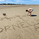6476 뷰
I may not be able to spend much time on the harmonic patterns and realized that there are not much open source scripts on them either. Hence, decided to release open source version which can be used by other developers for reference and build things on top of it.

Original script is protected and can be found here:

Logical ratios of patterns are coded as below:

Notations:
• Lines XABCD forms the pattern in all cases. (OXABCD in case of Three drives )
• abc = BC retacement of AB, xab = AB retracement of XA and so on

ABCD Classic
• 0.618 <= abc <= 0.786
• 1.272 <= bcd <= 1.618

AB=CD
• Price difference between AB and CD are equal
• Time difference between AB and CD are equal

ABCD Extension
• 0.618 <= abc <= 0.786
• 1.272 <= AD/ BC (price) <= 1.618

Gartley
• xab = 0.618
• 0.382 <= abc <= 0.886
• 1.272 <= bcd <= 1.618 OR xad = 0.786

Crab
• 0.382 <= xab <= 0.618
• 0.382 <= abc <= 0.886
• 2.24 <= bcd <= 3.618 OR xad = 1.618

Deep Crab
• xab = 0.886
• 0.382 <= abc <= 0.886
• 2.0 <= bcd <= 3.618 OR xad = 1.618

Bat
• 0.382 <= xab <= 0.50
• 0.382 <= abc <= 0.886
• 1.618 <= bcd <= 2.618 OR xad = 0.886

Butterfly
• xab = 0.786
• 0.382 <= abc <= 0.886
• 1.618 <= bcd <= 2.618 OR 1.272 <= xad <= 2.618

Shark
• xab = 0.786
• 1.13 <= abc <= 1.618
• 1.618 <= bcd <= 2.24 OR 0.886 <= xad <= 1.13

Cypher
• 0.382 <= xab <= 0.618
• 1.13 <= abc <= 1.414
• 1.272 <= bcd <= 2.0 OR xad = 0.786

Three Drives
• oxa = 0.618
• 1.27 <= xab <= 1.618
• abc = 0.618
• 1.27 <= bcd <= 1.618

5-0
• 1.13 <= xab <= 1.618
• 1.618 <= abc <= 2.24
• bcd = 0.5

This script contains everything which original script has apart from stats. Use the original script if you are not developer looking for code reference and prefer having stats table.

I have also developed a strategy based on harmonic patterns which can be found here:
릴리즈 노트:
• Removed deviation threshold and disable stats on load. (Can be enabled in settings)
• Default length is set to 5
릴리즈 노트: Update labels
릴리즈 노트: update labels
릴리즈 노트: Fix error
릴리즈 노트: Remove stats again
릴리즈 노트: Corrected errors in 3-Drive and 5-0 where B does not need to be in the middle.
릴리즈 노트: Minor correction in zigzag calculation logic.

Also, check my other script on harmonic pattern (Again - open source)
오픈 소스 스크립트

진정한 TradingView의 정신에 따라, 이 스크립트의 작성자는 스크립트를 오픈소스로 게시했기에 거래자들이 이해하고 확인할 수 있습니다. 작가님께 건배! 스크립트를 무료로 사용할 수 있지만, 게시물에서 이 코드를 재사용하는 것은 하우스룰을 따릅니다. 당신은 스크립트를 차트에 사용하기 위해 그것을 즐겨찾기 할 수 있습니다.

차트에 이 스크립트를 사용하시겠습니까?

## 코멘트Ankit_1618
@Ankit_1618, Thanks very much :) There are few other harmonic pattern variations I have published after this. Have a look. Also have a look at Auto Harmonic Patterns - V2 - which is protected script. But, you will still be able to use it.
응답Really useful for my trading style.
A huge thanks Bro !!
응답